# The book

The book has 280 pages. Each side is a rectangle with sides 15 cm and 22 cm.

a) how many leaves has the book?
b) at least how many square meters of paper should be used to production this book?

a =  140
b =  4.62 m2

### Step-by-step explanation:

$a=280\mathrm{/}2=140$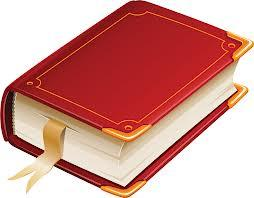Did you find an error or inaccuracy? Feel free to write us. Thank you!Tips to related online calculators
Do you want to convert area units?
Do you want to convert length units?

#### You need to know the following knowledge to solve this word math problem:

We encourage you to watch this tutorial video on this math problem:

## Related math problems and questions:

• Two sides paintThe door has the shape of a rectangle with dimensions of 260cm and 170cm. How many cans of paint will be needed to paint this door if one can of paint cover 2m2 of the area? We paint the doors on both sides.
• TailorTailor has an estimated 11 meters of 80 cm wide fabric. He will shape wholly 7 rectangles measuring 80 x 150 cm and nothing fabric does not remain. How many fabric he have?
• TableclothsThe restaurant has sixty-two square tablecloths with a side length of 150 cm and 36 rectangular tablecloths with dimensions of 140 cm and 160 cm. A) How many meters of hemming ribbon will be needed if we add 50 cm to each tablecloth? B) The ribbon sale in
• Pavilion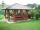The rectangular pavilion with dimensions 3.5 m and 2.75 m to be paved with square tiles of side 25 cm price of CZK 22 per 1 piece or rectangular tiles with sides of 20 cm and 15 cm in the price of CZK 11 per 1 pc. Which solution is cheaper (write its pric
• RectanglesHow many different rectangles with sides integers (in mm) have a circumference exactly 1000 cm? (a rectangle with sides of 50cm and 450cm is considered to be the same as a rectangle with sides of 450cm and 50cm)
• Table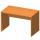Find the circumference of the table, where the long side is 1.28 meters and the short side is 86 cm.
• Do you solve this?Determine area S of rectangle and length of its sides if its perimeter is 102 cm.
• Christmas napkinsThe girls embroidered Christmas napkins. Each napkin had the shape of a triangle with sides of 5 dm, 60 cm, and 800 mm. How many cms did the girls sew if they made 15 napkins?
• Square gridSquare grid consists of a square with sides of length 1 cm. Draw in it at least three different patterns such that each had a content of 6 cm2 and circumference 12 cm and that their sides is in square grid.
• Paper boxCalculate whether 11 dm² of paper is sufficient for gluing a box without a lid with bottom dimensions of 2 dm and 15 cm and 12 cm high. Write result as: 0 = No, 1 = Yes
• Flooring a roomFind the cost of flooring a room 6.5 m by 5 m with square tiles of sides 25 cm at the rate of rupees 9.40 per tile.
• Two 2D shapes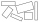Decide which shapes have more area:   (a) a square of 8cm side; or   (b) two rectangles with sides 5cm and 15cm? Write result as 1 or 2 (rectangles)
• The corridor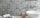The corridor is 12 m long and 3.6 m wide. It must be paved with rectangular tiles measuring 15 cm and 30 cm. Are 1000 pieces of tiles enough to pave the corridor?
• Book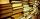Jitka read on holidays book that has 180 pages. In the first week read 45 pages. In the second week she read 15 pages more than the first week. How many pages left to read it yet?
• Painter 3Dad want to paint wall high 250 cm wide and 230 cm with wallpaper. How many meters must buy wallpaper if wallpaper width is 60 cm?
• Strip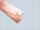From 5.9 cm wide strip should be cut rhombus with area 28 cm2. How long will be its side?
• SquaresCalculate the area of square and result round to square decimeters. a) a = 1,52 dm b) a = 13 268mm c) a = 562 cm d) a = 1,52 m